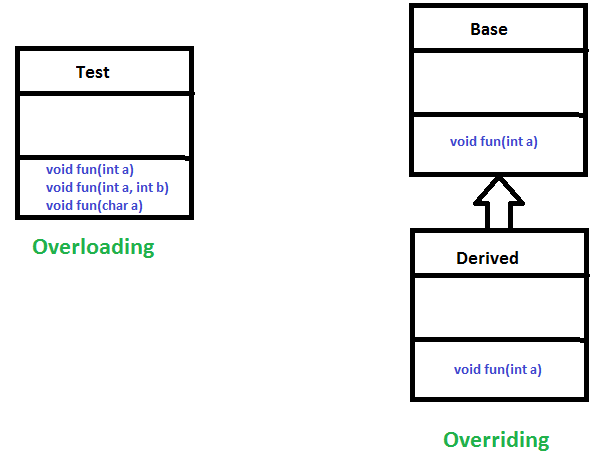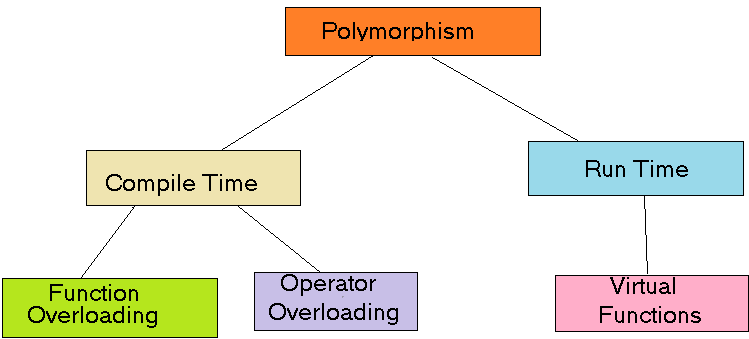GeeksforGeeks App
Open AppBrowser
Continue

## Related Articles

• Write an Interview Experience
• Java Tutorial
• Java Collection Tutorial

# Polymorphism in Java

The word polymorphism means having many forms. In simple words, we can define polymorphism as the ability of a message to be displayed in more than one form.

Real-life Illustration Polymorphism: A person at the same time can have different characteristics. Like a man at the same time is a father, a husband, and an employee. So the same person possesses different behavior in different situations. This is called polymorphism.

## What is Polymorphism in Java?

Polymorphism is considered one of the important features of Object-Oriented Programming. Polymorphism allows us to perform a single action in different ways. In other words, polymorphism allows you to define one interface and have multiple implementations. The word “poly” means many and “morphs” means forms, So it means many forms.

## Types of Java polymorphism

In Java polymorphism is mainly divided into two types:

• Compile-time Polymorphism
• Runtime Polymorphism

## Compile-Time PolymorphismWhen there are multiple functions with the same name but different parameters then these functions are said to be overloaded. Functions can be overloaded by changes in the number of arguments or/and a change in the type of arguments.

Example 1:

## Java

 `// Java Program for Method overloading``// By using Different Types of Arguments ` `// Class 1``// Helper class``class` `Helper {` `    ``// Method with 2 integer parameters``    ``static` `int` `Multiply(``int` `a, ``int` `b)``    ``{` `        ``// Returns product of integer numbers``        ``return` `a * b;``    ``}` `    ``// Method 2``    ``// With same name but with 2 double parameters``    ``static` `double` `Multiply(``double` `a, ``double` `b)``    ``{` `        ``// Returns product of double numbers``        ``return` `a * b;``    ``}``}` `// Class 2``// Main class``class` `GFG {` `    ``// Main driver method``    ``public` `static` `void` `main(String[] args)``    ``{` `        ``// Calling method by passing``        ``// input as in arguments``        ``System.out.println(Helper.Multiply(``2``, ``4``));``        ``System.out.println(Helper.Multiply(``5.5``, ``6.3``));``    ``}``}`

Output

```8
34.65```

Example 2:

## Java

 `// Java program for Method Overloading``// by Using Different Numbers of Arguments` `// Class 1``// Helper class``class` `Helper {` `    ``// Method 1``    ``// Multiplication of 2 numbers``    ``static` `int` `Multiply(``int` `a, ``int` `b)``    ``{` `        ``// Return product``        ``return` `a * b;``    ``}` `    ``// Method 2``    ``// // Multiplication of 3 numbers``    ``static` `int` `Multiply(``int` `a, ``int` `b, ``int` `c)``    ``{` `        ``// Return product``        ``return` `a * b * c;``    ``}``}` `// Class 2``// Main class``class` `GFG {` `    ``// Main driver method``    ``public` `static` `void` `main(String[] args)``    ``{` `        ``// Calling method by passing``        ``// input as in arguments``        ``System.out.println(Helper.Multiply(``2``, ``4``));``        ``System.out.println(Helper.Multiply(``2``, ``7``, ``3``));``    ``}``}`

Output

```8
42```

## Subtypes of Compile-time Polymorphism:

It is a feature in C++ where multiple functions can have the same name but with different parameter lists. The compiler will decide which function to call based on the number and types of arguments passed to the function.

It is a feature in C++ where the operators such as +, -, *, etc. can be given additional meanings when applied to user-defined data types.

### iii. Template

it is a powerful feature in C++ that allows us to write generic functions and classes. A template is a blueprint for creating a family of functions or classes.

## Runtime Polymorphism

It is also known as Dynamic Method Dispatch. It is a process in which a function call to the overridden method is resolved at Runtime. This type of polymorphism is achieved by Method Overriding. Method overriding, on the other hand, occurs when a derived class has a definition for one of the member functions of the base class. That base function is said to be overridden.

Example

## Java

 `// Java Program for Method Overriding` `// Class 1``// Helper class``class` `Parent {` `    ``// Method of parent class``    ``void` `Print()``    ``{` `        ``// Print statement``        ``System.out.println(``"parent class"``);``    ``}``}` `// Class 2``// Helper class``class` `subclass1 ``extends` `Parent {` `    ``// Method``    ``void` `Print() { System.out.println(``"subclass1"``); }``}` `// Class 3``// Helper class``class` `subclass2 ``extends` `Parent {` `    ``// Method``    ``void` `Print()``    ``{` `        ``// Print statement``        ``System.out.println(``"subclass2"``);``    ``}``}` `// Class 4``// Main class``class` `GFG {` `    ``// Main driver method``    ``public` `static` `void` `main(String[] args)``    ``{` `        ``// Creating object of class 1``        ``Parent a;` `        ``// Now we will be calling print methods``        ``// inside main() method` `        ``a = ``new` `subclass1();``        ``a.Print();` `        ``a = ``new` `subclass2();``        ``a.Print();``    ``}``}`

Output

```subclass1
subclass2```

#### Explanation of the above code:

Here in this program, When an object of a child class is created, then the method inside the child class is called. This is because The method in the parent class is overridden by the child class. Since The method is overridden, This method has more priority than the parent method inside the child class. So, the body inside the child class is executed.

## Subtype of Run-time Polymorphism

### i. Virtual functions

It allows an object of a derived class to behave as if it were an object of the base class. The derived class can override the virtual function of the base class to provide its own implementation. The function call is resolved at runtime, depending on the actual type of the object.

Diagram –Polymorphism in Java is a concept that allows objects of different classes to be treated as objects of a common class. It enables objects to behave differently based on their specific class type.

### Advantages of Polymorphism in Java

1. Increases code reusability by allowing objects of different classes to be treated as objects of a common class.
2. Improves readability and maintainability of code by reducing the amount of code that needs to be written and maintained.
3. Supports dynamic binding, enabling the correct method to be called at runtime, based on the actual class of the object.
4. Enables objects to be treated as a single type, making it easier to write generic code that can handle objects of different types.

### Disadvantages of Polymorphism in Java

1. Can make it more difficult to understand the behavior of an object, especially if the code is complex.
2. This may lead to performance issues, as polymorphic behavior may require additional computations at runtime.

My Personal Notes arrow_drop_up